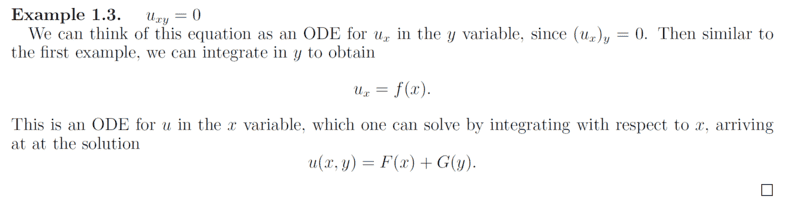# Solve the Partial differential equation ##U_{xy}=0##

• A
• chwala
This is a common notational convention in mathematics.In summary, the conversation discusses integrating the equation ##U_{xy}=0## with respect to y and x, leading to the conclusion that the solution is of the form ##U(x,y)=F(x)+G(y)##. The conversation also clarifies the use of notation in partial derivative equations.

#### chwala

Gold Member
TL;DR Summary
I am going through these notes; i want to check that i am gettting it right...

Solve the pde;

##U_{xy}=0##
This is part of the notes;My own way of thought;

Given;

##U_{xy}=0##

then considering ##U_x## as on ode in the ##y## variable; we integrate both sides with respect to ##y## i.e

##\dfrac{du}{dx} \int \dfrac{1}{dy} dy=\int 0 dy##

this is the part i need insight...the original problem involves partial derivatives but in this case when we integrate with respect to ##y## we are integrating with respect to ##dy## and not ##∂y## ...correct?

##U_x= 0 + k##, where ##k## is a constant in terms of ##x## therefore,

##U_x= f(x)##, an arbitrary function of ##x##... which is an ode for ##u## in the ##x## variable. On integrating again with respect to ##x## we get,

##U(x,y) = F(x) + k##, where ##k## is a constant in terms of ##y## therefore,

##U(x,y) = F(x) + H(y)##

cheers!

Last edited:
I interpret you as follows.
$$\frac{\partial^2 U(x,y)}{\partial x \partial y}=0$$
Integration by y with x=const. or by x with y=const.
$$\frac{\partial U}{\partial x }=f(x)$$
$$\frac{\partial U}{\partial y }=g(y)$$
Thus
$$dU=f(x)dx+g(y)dy$$
$$U(x,y)=F(x)+G(y)$$

Last edited:
•chwala
anuttarasammyak said:
I interpret you as follows.
$$\frac{\partial^2 U(x,y)}{\partial x \partial y}=0$$
Integration by y with x=const. or by x with y=const.
$$\frac{\partial U}{\partial x }=f(x)$$
$$\frac{\partial U}{\partial y }=g(y)$$
Thus
$$dU=f(x)dx+g(y)dy$$
$$U(x,y)=F(x)+G(y)$$
Is there another way bro? ...they call it method of characteristic ...need to read on this...

chwala said:
Given;
##U_{xy}=0##

then considering ##U_x## as on ode in the ##y## variable; we integrate both sides with respect to ##y## i.e

##\dfrac{du}{dx} \int \dfrac{1}{dy} dy=\int 0 dy##
The above doesn't make any sense to me. Clearly the last integral would be 0 + a constant.
chwala said:
this is the part i need insight...the original problem involves partial derivatives but in this case when we integrate with respect to ##y## we are integrating with respect to ##dy## and not ##∂y## ...correct?
The expression ##dy## serves only to indicate the variable with respect to which integration is taking place. You are integrating with respect to y, not dy, and definitely not with respect to ∂y.

•chwala